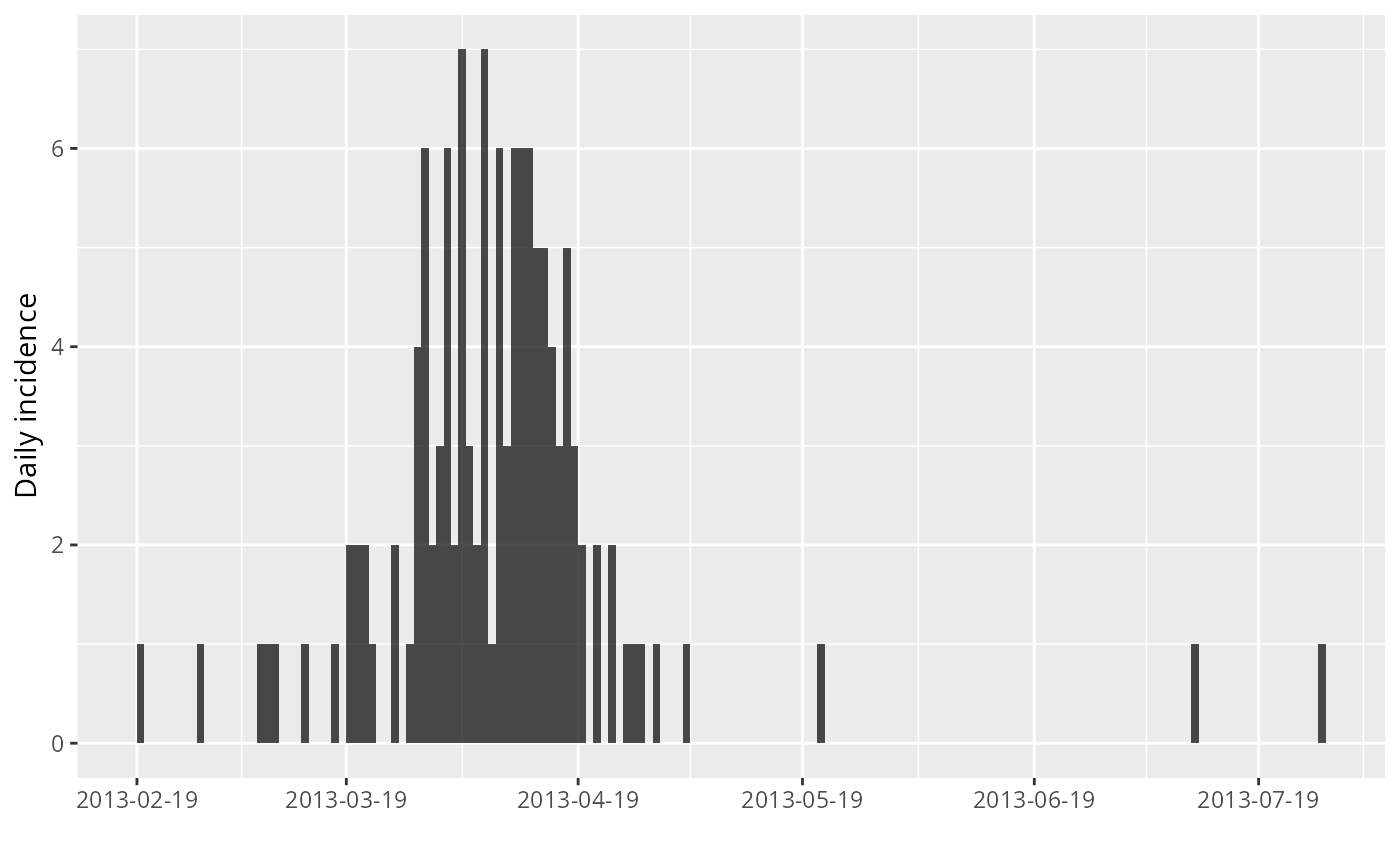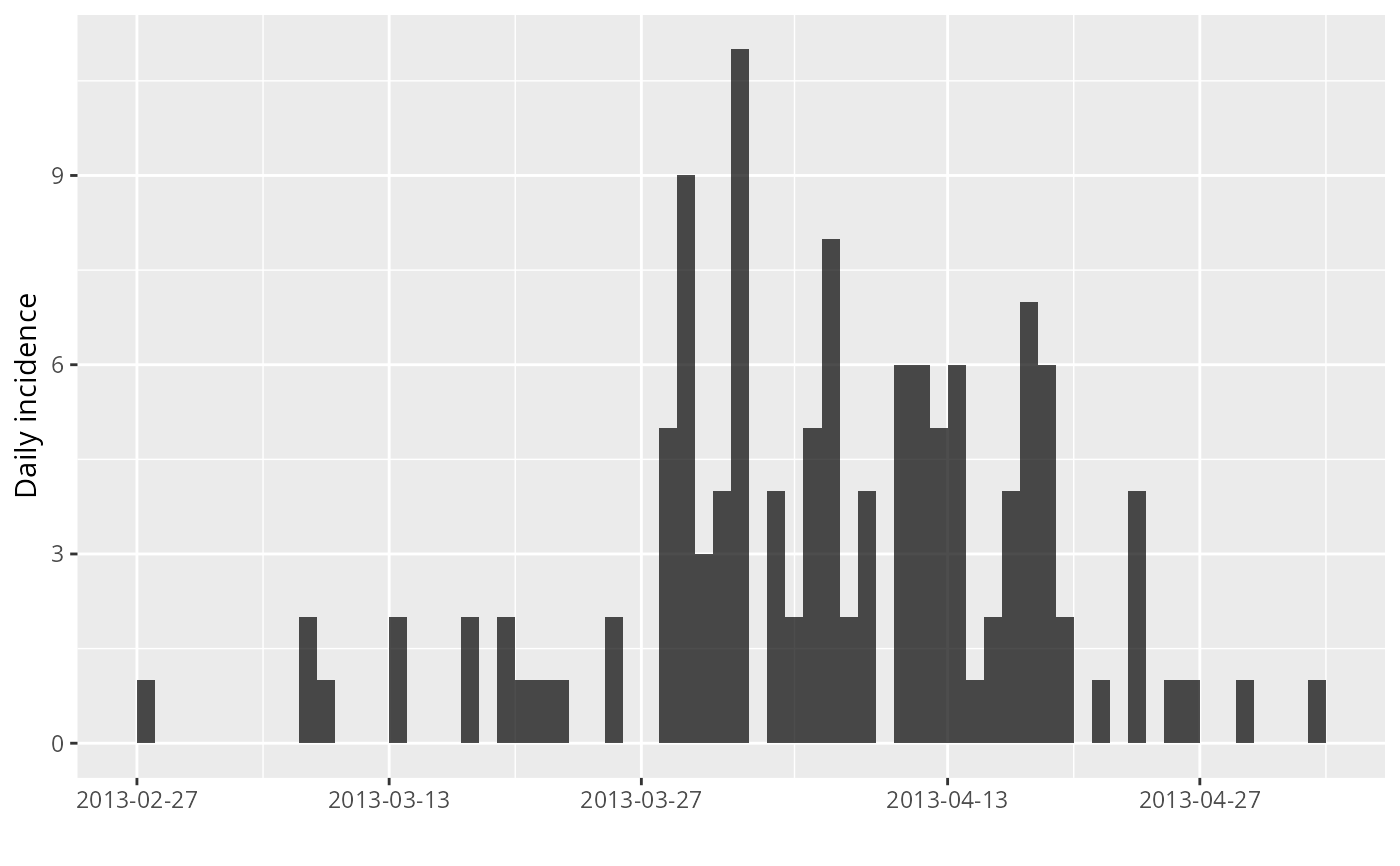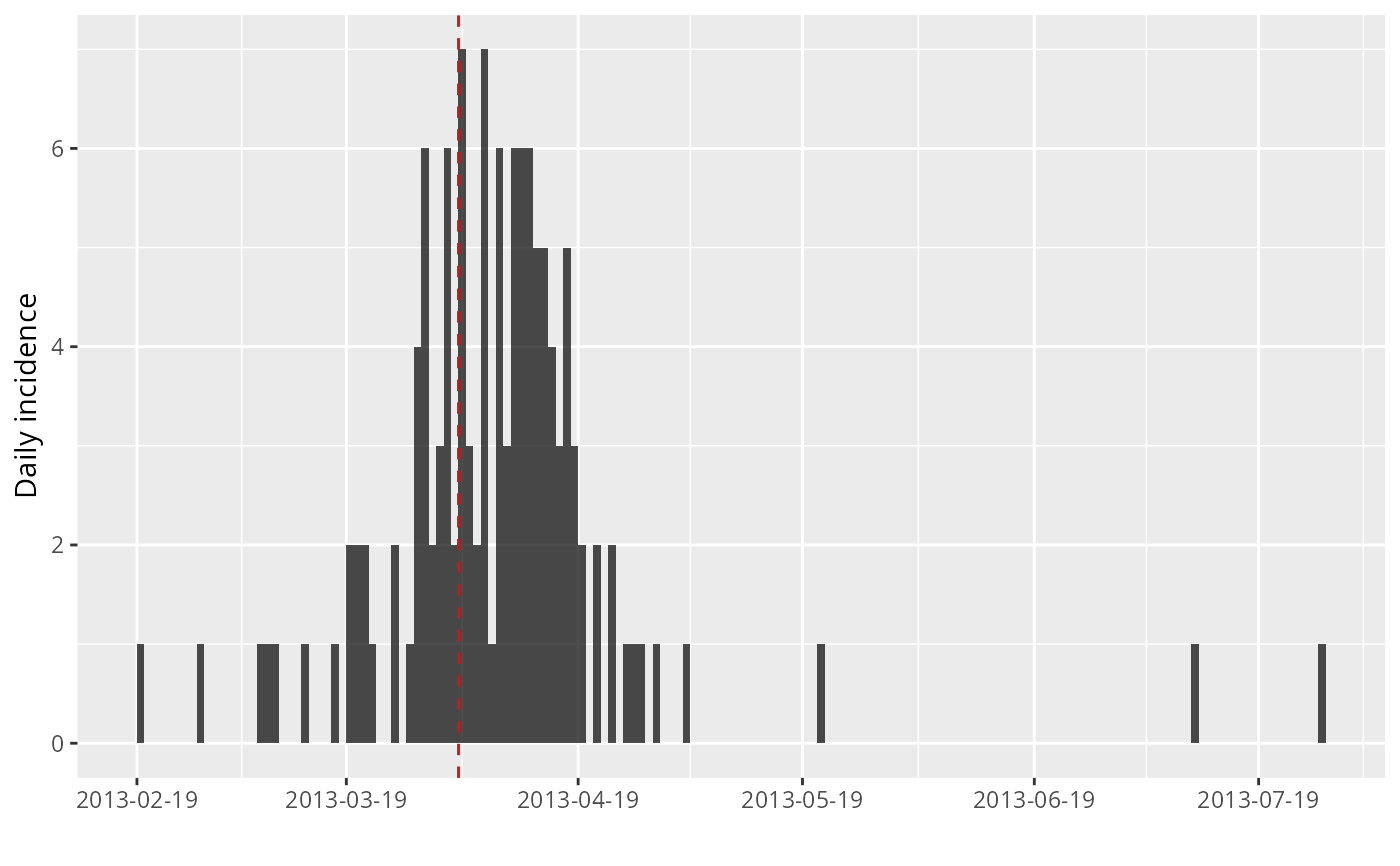This function can be used to find the peak of an epidemic curve stored as an incidence object.

find_peak(x, pool = TRUE)

## Arguments

x An incidence object. If TRUE (default), any groups will be pooled before finding a peak. If FALSE, separate peaks will be found for each group.

## Value

The date of the (first) highest incidence in the data.

estimate_peak() for bootstrap estimates of the peak time

## Examples


if (require(outbreaks) && require(ggplot2)) { withAutoprint({
i <- incidence(fluH7N9_china_2013$date_of_onset) i plot(i) ## one simple bootstrap x <- bootstrap(i) x plot(x) ## find 95% CI for peak time using bootstrap find_peak(i) ## show confidence interval plot(i) + geom_vline(xintercept = find_peak(i), col = "red", lty = 2) })}#> > i <- incidence(fluH7N9_china_2013$date_of_onset)#> 10 missing observations were removed.#> > i
#> <incidence object>
#> [126 cases from days 2013-02-19 to 2013-07-27]
#>
#> $counts: matrix with 159 rows and 1 columns #>$n: 126 cases in total
#> $dates: 159 dates marking the left-side of bins #>$interval: 1 day
#> $timespan: 159 days #>$cumulative: FALSE
#>
#> > plot(i)#> > x <- bootstrap(i)
#> > x
#> <incidence object>
#> [126 cases from days 2013-02-27 to 2013-05-03]
#>
#> $counts: matrix with 66 rows and 1 columns #>$n: 126 cases in total
#> $dates: 66 dates marking the left-side of bins #>$interval: 1 day
#> $timespan: 66 days #>$cumulative: FALSE
#>
#> > plot(x)#> > find_peak(i)
#>  "2013-04-03"
#> > plot(i) + geom_vline(xintercept = find_peak(i), col = "red", lty = 2)